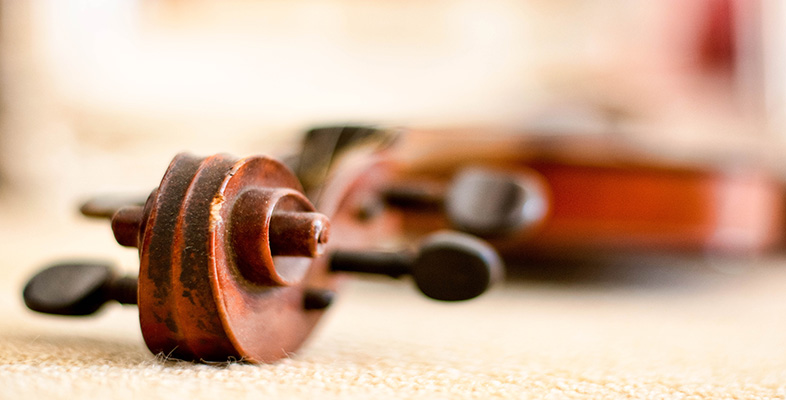Creating musical sounds

This free course is available to start right now. Review the full course description and key learning outcomes and create an account and enrol if you want a free statement of participation.

Free course

# 5.9 Vibrating air column: standing waves in a cylindrical tube closed at one end

We'll now turn our attention to the setting up of standing waves in an air column contained within a cylindrical tube that is open at one end but closed at the other. Straight away we can say that the closed end must be a displacement node since the air molecules can't move at this boundary. That means it must be a pressure antinode. The open end, as we saw previously, will be a displacement antinode (that is, a pressure node).

Now, you may recall that the distance between a node and a neighbouring antinode is a quarter of a wavelength (look back at the discussion preceding Activity 9 if you are unsure). More to the point, as is clear from a careful look at Figure 7, the distance between a node and any arbitrary antinode must be an odd number of quarter wavelengths. Therefore, the air column must be either one quarter wavelength long, three quarter wavelengths long, five quarter wavelengths long, seven quarter wavelengths long and so on. If the length of the air column is denoted by L and the wavelength by λ then this can be expressed in the following way: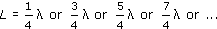Since we can use the expression (2n − 1), where n = 1, 2, 3, 4, …, to represent the series of odd numbers (try it out to satisfy yourself), this can be written more concisely as: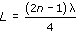Rearranging this equation, we can show that the wavelengths of standing waves that can be set up in the air column are therefore given by: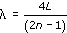Remembering that the frequency of a sound wave is related to its wavelength by the expression f = v/λ, where v is the speed of sound, the resonance frequencies of an air column that is closed at one end can be written: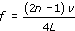The normal modes of vibration plotted in terms of pressure are shown in Figure 16. Again, the solid curve shows the pressure at one extreme of the cycle and the dashed curve shows the pressure one half-cycle later. The separation between the curved lines at any position along the tube represents the pressure amplitude at that position.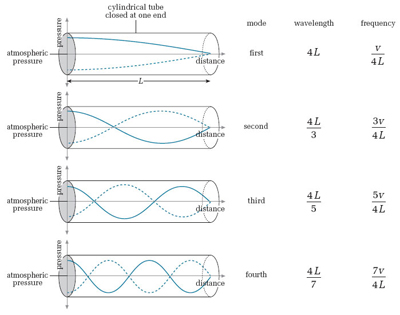Figure 16 The first four normal modes of vibration for a cylindrical tube closed at one end, plotted in terms of pressure. The solid curve shows the pressure at one extreme of the cycle and the dashed curve shows the pressure one half-cycle later

In the first mode (n = 1) there is a pressure antinode at the closed end and a pressure node at the open end. There are no other nodes or antinodes within the air column. The frequency at which this standing-wave pattern is set up is f = v/4L.

In the second mode (n = 2) again there is a pressure antinode at the closed end and a pressure node at the open end. However, there is now another pressure node a third of the way along the air column from the closed end and another pressure antinode two thirds of the way along. The frequency at which this standing-wave pattern is set up is f = 3v/4L

In the third mode (n = 3) there is a pressure antinode at the closed end, a pressure node at the open end, and two more pressure nodes and two more pressure antinodes within the air column. The frequency at which this standing-wave pattern is set up is f = 5v/4L.

If the fundamental frequency of the air column is denoted by f1, then the frequencies at which the second, third, fourth and later modes occur are 3f1, 5f1, 7f1 …, (2n − 1)f1. The resonance frequencies don't form a complete harmonic series. Only the odd harmonics are present.

## Activity 19

Calculate the first three resonance frequencies of a 1.5 m long cylindrical tube open at one end and closed at the other. Assume the speed of sound is 340 m/s.

### Answer

If the cylinder is closed at one end, then the formula that gives the resonance frequencies is:where L is the length of the cylinder and v is the speed of sound.

If L = 1.5 m and v = 340 m/s, the first three resonance frequencies are determined as follows:

First resonance frequency f1 = v/4L = 340/(4 × 1.5) = 56.7 Hz.

Second resonance frequency f2 = 3v/4L = (3 × 340)/(4 × 1.5) = 170 Hz.

Third resonance frequency f3 = 5v/4L = (5 × 340)/(4 × 1.5) = 283.3 Hz.

TA212_2

### Take your learning further

Making the decision to study can be a big step, which is why you'll want a trusted University. The Open University has 50 years’ experience delivering flexible learning and 170,000 students are studying with us right now. Take a look at all Open University courses.

If you are new to university level study, find out more about the types of qualifications we offer, including our entry level Access courses and Certificates.

Not ready for University study then browse over 900 free courses on OpenLearn and sign up to our newsletter to hear about new free courses as they are released.

Every year, thousands of students decide to study with The Open University. With over 120 qualifications, we’ve got the right course for you.

Request an Open University prospectus